## Saturday, 9 August 2014

### CHAPTER 2 - Section Formula

Consider two arbitrary points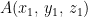$A({x_1},\,{y_1},\,{z_1})$ and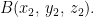$B({x_2},\,{y_2},\,{z_2}).$ We need to find the coordinates of the points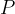$P$ and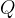$Q$ dividing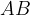$AB$ internally and externally respectively, in the ratio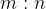$m : n$.
The approach used in the evaluation of the coordinates of$P$ and$Q$ is analogous to how we derived the section formula in the two dimensional case.In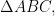$\Delta ABC,$ we have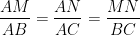$\dfrac{{AM}}{{AB}} = \dfrac{{AN}}{{AC}} = \dfrac{{MN}}{{BC}}$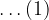$\ldots(1)$
Assume the coordinates of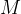$M$ to be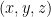$(x,y,z)$. Thus, the relation in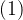$(1)$ can be written as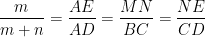$\dfrac{m}{{m + n}} = \dfrac{{AE}}{{AD}} = \dfrac{{MN}}{{BC}} = \dfrac{{NE}}{{CD}}$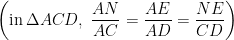$\left( {{\rm{in }}\,\Delta ACD,\,\,\dfrac{{AN}}{{AC}} = \dfrac{{AE}}{{AD}} = \dfrac{{NE}}{{CD}}} \right)$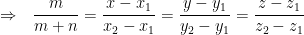$\Rightarrow \,\,\,\, \dfrac{m}{{m + n}} = \dfrac{{x - {x_1}}}{{{x_2} - {x_1}}} = \dfrac{{y - {y_1}}}{{{y_2} - {y_1}}} = \dfrac{{z - {z_1}}}{{{z_2} - {z_1}}}$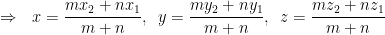$\Rightarrow \,\,\,\, x = \dfrac{{m{x_2} + n{x_1}}}{{m + n}},\,\,\,y = \dfrac{{m{y_2} + n{y_1}}}{{m + n}},\,\,\,z = \dfrac{{m{z_2} + n{z_1}}}{{m + n}}$
Thus, the coordinates of$M$ are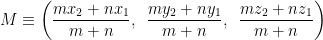${M \equiv \left( {\dfrac{{m{x_2} + n{x_1}}}{{m + n}},\,\,\,\dfrac{{m{y_2} + n{y_1}}}{{m + n}},\,\,\,\dfrac{{m{z_2} + n{z_1}}}{{m + n}}} \right)}$
The form of the coordinates is the same as in the two dimensional case, as might have been expected. The coordinates of$M$‘ which divides$AB$ externally in the ratio$m : n$ can be obtained by substituting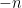$- n$ for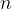$n$ in the coordinates of$M$.
As elementary applications of the section formula, do the following problems :
 (a) The mid-points of the sides of a triangle are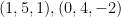$(1,5,1),(0,4, - 2)$and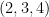$(2,3,4)$. Find its vertices. (b) Find the coordinates of the centroid of the triangle with vertices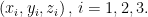$\left( {{x_i},{y_i},{z_i}} \right),\,i = 1,2,3.$

#### DIRECTION COSINES AND DIRECTION RATIOS

The direction cosines of a (directed) line are the cosines of the angles which the line makes with the positive directions of the coordinate axes.
Consider a line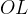$OL$ as shown, passing through the origin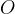$O$. Let$OL$ be inclined at angles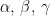$\alpha ,\,\beta ,\,\gamma$ to the coordinate axes.Thus, the direction cosines are given by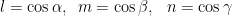$l = \cos \alpha ,\,\,\,m = \cos \beta ,\,\,\,\,n = \cos \gamma$
Note that for the line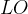$LO$ (i.e., the directed line segment in the direction opposite to$OL$), the direction cosines will be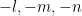$- l, - m, - n$.
The direction cosines for a directed line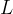$L$ not passing through the origin are the same as the direction cosines of the directed line parallel to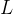$L$ and passing through the origin.
Note that for any point$P$ lying on the line$OL$ with direction cosines$l$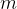$m$$n$such that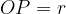$OP = r$, the coordinates of$P$ will be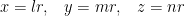$x = lr,\,\,\,\,\,y = mr,\,\,\,\,\,z = nr$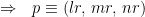$\Rightarrow \,\,\,\, p \equiv \left( {lr,\,mr,\,nr} \right)$
Now, since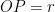$OP = r$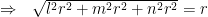$\Rightarrow \,\,\,\, \sqrt {{l^2}{r^2} + {m^2}{r^2} + {n^2}{r^2}} = r$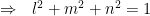$\Rightarrow\,\,\,\,{{l^2} + {m^2} + {n^2} = 1}$
The direction cosines of any line will satisfy this relation.
The direction ratios are simply a set of three real numbers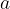$a$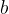$b$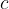$c$ proportional to$l$$m$$n$, i.e.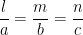$\dfrac{l}{a} = \dfrac{m}{b} = \dfrac{n}{c}$
From this relation, we can write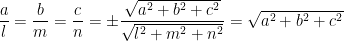$\dfrac{a}{l} = \dfrac{b}{m} = \dfrac{c}{n} = \pm \dfrac{{\sqrt {{a^2} + {b^2} + {c^2}} }}{{\sqrt {{l^2} + {m^2} + {n^2}} }} = \sqrt {{a^2} + {b^2} + {c^2}}$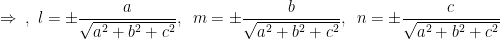$\Rightarrow\,\,\,,\,\,{l = \pm \dfrac{a}{{\sqrt {{a^2} + {b^2} + {c^2}} }},\,\,\,m = \pm \dfrac{b}{{\sqrt {{a^2} + {b^2} + {c^2}} }},\,\,\,n = \pm \dfrac{c}{{\sqrt {{a^2} + {b^2} + {c^2}} }}}$
These relations tell us how to find the direction cosines from direction ratios.
Note that the direction cosines for any line must be unique. However, there are infinitely many sets of direction ratios since direction ratios are just a set of any three numbers proportional to the direction cosines.